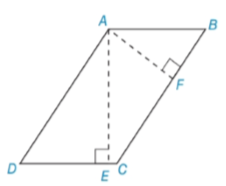Chapter 5.3, Problem 38EElementary Geometry For College St...

7th Edition
Alexander + 2 others
ISBN: 9781337614085

Solutions

Chapter
SectionElementary Geometry For College St...

7th Edition
Alexander + 2 others
ISBN: 9781337614085
Textbook Problem

Use the result of Exercise 13 to do the following problem. In ▱ A B C D , A B = 7 and B C = 12 . The length of altitude A F ¯ (to side B C ¯ ) is 5. Find the length of altitude A E ¯ from A to D C ¯ .To determine

To find:

The length of altitude AE¯ from A to DC¯ by using the result of Exercise 13.

Explanation

Given:

Given: ABCD, AB=7, BC=12, and the length of altitude AF¯ (to side BC¯) is 5.

Definition:

AA:

If the two angles of one triangle are congruent to the two angles of another triangle, then the triangles are similar.

CSSTP:

Corresponding sides of similar triangles are proportional.

Description:

Given that MNPQ,.

From the given figure, it is observed that there are two altitudes AE¯ and AF¯.

An altitude is a line segment through a vertex and perpendicular to a line of a base.

That is, AE¯DC¯ and AF¯BC¯.

It is known that two lines are perpendicular, they form right angles. That is, AED and AFB are right triangles.

Since all right angles are congruent, AEDAFB.

The opposite angles of are congruent. So that DB.

The above mentioned AA definition, the two triangles AED and AFB are similar since the two angles of one triangle are congruent to two angles of another triangle

Still sussing out bartleby?

Check out a sample textbook solution.

See a sample solution

The Solution to Your Study Problems

Bartleby provides explanations to thousands of textbook problems written by our experts, many with advanced degrees!

Get Started

2x2 + 8x + 7 = 0

Applied Calculus for the Managerial, Life, and Social Sciences: A Brief Approach

38.

Mathematical Applications for the Management, Life, and Social Sciences

Convert to whole or mixed numbers. 4.

Contemporary Mathematics for Business & Consumers

Given that for all x, a power series for is:

Study Guide for Stewart's Single Variable Calculus: Early Transcendentals, 8th

True or False: Let . Then f(x, y) is a joint probability function.

Study Guide for Stewart's Multivariable Calculus, 8th